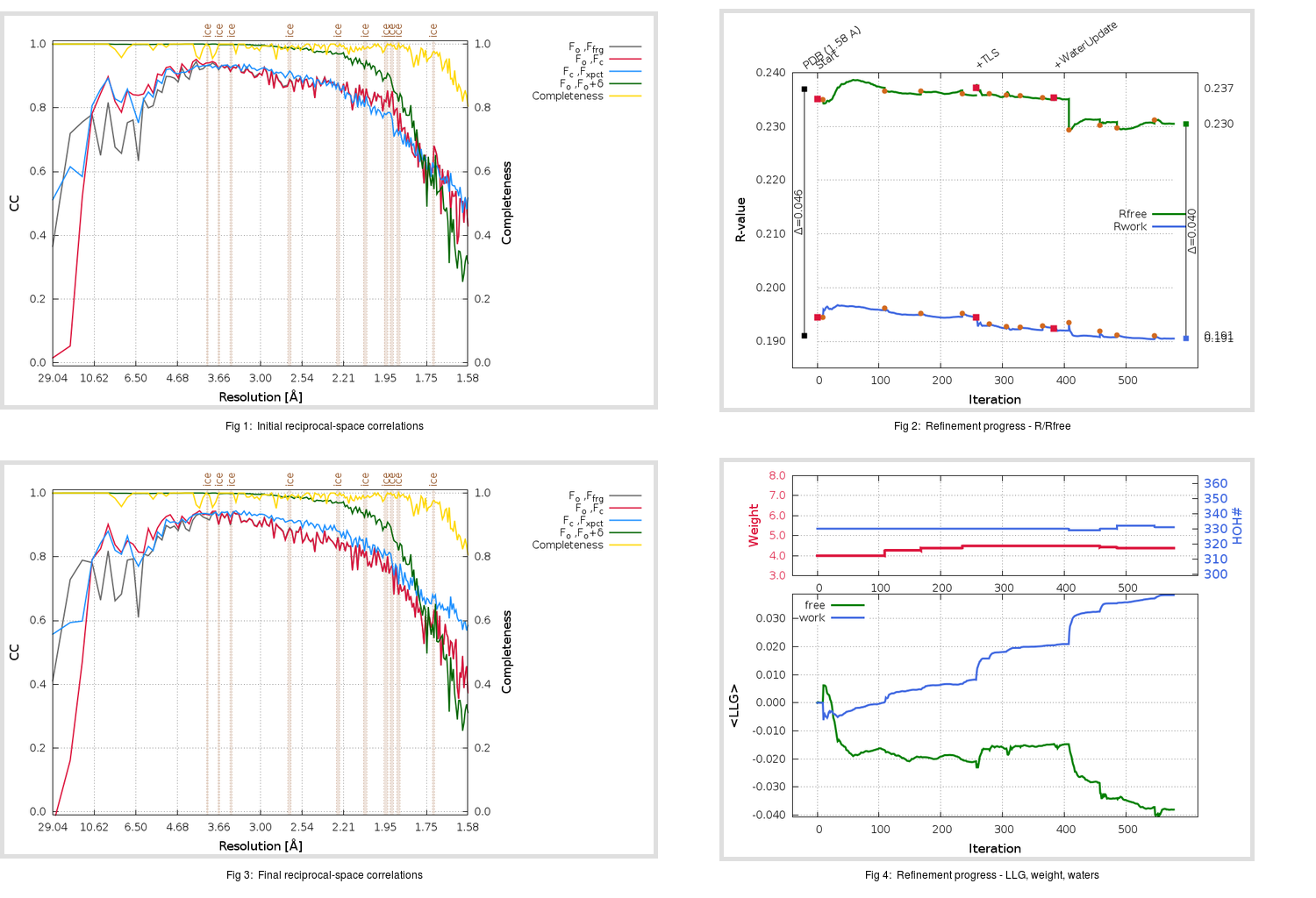Content:

## Deposited

` `
 Date deposited Date data collection Resolution R, Rfree 20200315 20200306 1.58 0.1890 0.2370

Molprobity (CCP4 7.0 version) summary:

```Ramachandran outliers =   0.66 %
favored =  97.68 %
Rotamer outliers      =   1.14 %
C-beta deviations     =     0
Clashscore            =   5.70
RMS(bonds)            =   0.0131
RMS(angles)           =   1.83
MolProbity score      =   1.42
Resolution            =   1.58
R-work                =   0.1890
R-free                =   0.2370
```

```Number of waters      =   330

<B> (all atoms) =   24.05 ( sd =    9.06 ) for       2728 non-hydrogen atoms
<B>   (protein) =   22.66 ( sd =    8.07 ) for       2370 non-hydrogen atoms
<B>     (water) =   33.32 ( sd =    9.45 ) for        330 non-hydrogen atoms
<B>    (others) =   30.68 ( sd =   14.21 ) for         28 non-hydrogen atoms

B min/max       (all non-hydrogen atoms) =   13.11 /   70.29
B min/max   (protein non-hydrogen atoms) =   13.11 /   70.29
B min/max     (water non-hydrogen atoms) =   14.41 /   64.06
B min/max     (other non-hydrogen atoms) =   17.75 /   44.46
```

## BUSTER (re-)refinement

` `

Molprobity (CCP4 7.0 version) summary:

```Ramachandran outliers =   0.66 %
favored =  98.34 %
Rotamer outliers      =   2.27 %
C-beta deviations     =     0
Clashscore            =   2.53
RMS(bonds)            =   0.0112
RMS(angles)           =   1.56
MolProbity score      =   1.31
Resolution            =   1.58
R-work                =   0.1906
R-free                =   0.2305
```

```Number of waters      =   331

<B> (all atoms) =   25.36 ( sd =    8.31 ) for       2729 non-hydrogen atoms
<B>   (protein) =   23.81 ( sd =    6.79 ) for       2370 non-hydrogen atoms
<B>     (water) =   35.70 ( sd =    9.54 ) for        331 non-hydrogen atoms
<B>    (others) =   32.92 ( sd =   15.27 ) for         28 non-hydrogen atoms

B min/max       (all non-hydrogen atoms) =    6.84 /   68.13
B min/max   (protein non-hydrogen atoms) =    6.84 /   54.80
B min/max     (water non-hydrogen atoms) =   16.12 /   68.13
B min/max     (other non-hydrogen atoms) =   20.33 /   46.37
```

Refinement progression:Results:

` `
 File Remark 5RFH_aB_refine.01_03_refine.pdb.gz exact refinement commands are in header 5RFH_aB_refine.01_03_refine.mtz.gz including original deposited data and several re-refinement map coefficients 5RFH_aB_refine.01_03_BUSTER_model.cif.gz including any non-standard compound restraints 5RFH_aB_refine.01_03_BUSTER_refln.cif.gz